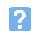## EAL Level 2 Engineering Science1. Which one of the following is the SI unit of mass?a) Pound (lb)b) kilogram (kg)c) gram (g)d) tonne (t)2. A voltage drop of 12V appears across a resistor of 24 ohm. Which one of the following gives the current flowing?a) 2Ab) 0.5Ac) 0.25Ad) 0.4A3. A force of 2N acts on a mass of 1.5kg. Which one of the following gives the resulting acceleration of the mass?a) 0.75 m/s2b) 1.5 m/s2c) 3 m/s2d) 1.33 m/s24. Which one of the following is the SI unit of elecric charge?a) Volt (V)b) Ampere (A)c) Coulomb (C)d) Farad (F)5. When a body is at rest (or in equilibrium), the action and reactionforces acting on it will:a) be equal and oppositeb) both be zeroc) produce an accelerationd) conteract gravity6. A light alloy beam has a mass of 25kg. Which one of the following gives the weight of the beam? (Take g = 9.81m/s/s).a) 2.54Nb) 25.Nc) 34.81Nd) 245.25N7. Which one of the following materials has the greatest density?a) Aluminiumb) Glassc) Mild steeld) Concrete8. A 'moment' is a froce thata) produces a turning effectb) cunteracts frictionc) restores equilibriumd) resists motion9. Which one of the following is the same as a temperature of 373K?a) 100 deg.Cb) 0 deg.Cc) 273 deg.Cd) 100 deg.F10. Which one of the following materials has the lowest coefficient of linear expansion?a) Aluminiumb) Brassc) Copperd) Invar11. Heat energy that is transferred in a liquid or gas due to motion of the liquid or gas from a warmer region to a cooler one is referred to as:a) Conductionb) Convectionc) Radiationd) Variation
This is more feedback!
This is the feedback!
feedback!